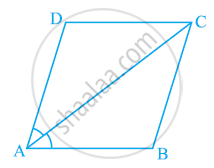# Diagonal AC of a parallelogram ABCD bisects ∠A (see the given figure). Show that (i) It bisects ∠C also, (ii) ABCD is a rhombus. - Mathematics

Diagonal AC of a parallelogram ABCD bisects ∠A (see the given figure). Show that

(i) It bisects ∠C also,

(ii) ABCD is a rhombus.#### Solution

(i) ABCD is a parallelogram.

∴ ∠DAC = ∠BCA (Alternate interior angles) ... (1)

And, ∠BAC = ∠DCA (Alternate interior angles) ... (2)

However, it is given that AC bisects ∠A.

∴ ∠DAC = ∠BAC ... (3)

From equations (1), (2), and (3), we obtain

∠DAC = ∠BCA = ∠BAC = ∠DCA ... (4)

⇒ ∠DCA = ∠BCA

Hence, AC bisects ∠C.

(ii)From equation (4), we obtain

∠DAC = ∠DCA

∴ DA = DC (Side opposite to equal angles are equal)

However, DA = BC and AB = CD (Opposite sides of a parallelogram)

∴ AB = BC = CD = DA

Hence, ABCD is a rhombus.

Concept: Another Condition for a Quadrilateral to Be a Parallelogram
Is there an error in this question or solution?

#### APPEARS IN

NCERT Class 9 Maths